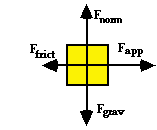# Mechanics: Newton's Laws of Motion

## Newton's Laws of Motion: Audio Guided Solution

#### Problem 11:Ethan is dragging a bag of grass from the garage to the street on the evening before garbage pick-up day. The diagram at the right is a free-body diagram. It uses arrows to represent the forces acting upon the bag. Each force is labeled according to type. The magnitude of the force is represented by the size of the arrow. Use the free body diagram to determine the net force acting upon the bag. The values of the individual forces are:

Fgrav = Fnorm = 60.5 N
Fapp = 40.2 N
Ffrict = 5.7 N.

## Habits of an Effective Problem Solver

• Read the problem carefully and develop a mental picture of the physical situation. If necessary, sketch a simple diagram of the physical situation to help you visualize it.
• Identify the known and unknown quantities in an organized manner. Equate given values to the symbols used to represent the corresponding quantity - e.g., vo = 0 m/s; a = 4.2 m/s/s; vf = 22.9 m/s; d = ???.
• Use physics formulas and conceptual reasoning to plot a strategy for solving for the unknown quantity.
• Identify the appropriate formula(s) to use.
• Perform substitutions and algebraic manipulations in order to solve for the unknown quantity.